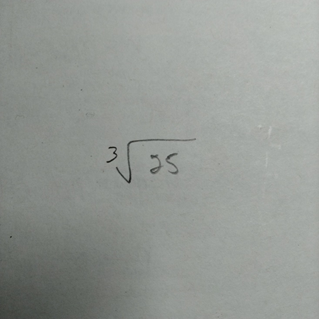## When Helping Means Winning contest

Ask a school-related question in the Help Zone and you could win one of three \$500 scholarships.

# Help Zone

### Student Question

Hi, what is the exponent of a square root like in the photo?

Thank you!Mathematics## Explanations (1)

•Explanation from AlloprofExplanation from Alloprof

This Explanation was submitted by a member of the Alloprof team.

Hello

To know the value of the exponent, I remind you of the following exponent law:

$$a^{\frac{m}{n}}=\sqrt[n]{a^m}$$

In your case, the exponent conversion of

$$\sqrt{25}$$

therefore becomes:

$$25^{\frac{1}{3}}$$

I suggest you to revise the exponent laws on the next page of our website:

Looking forward to answering more of your questions in the help zone! :)